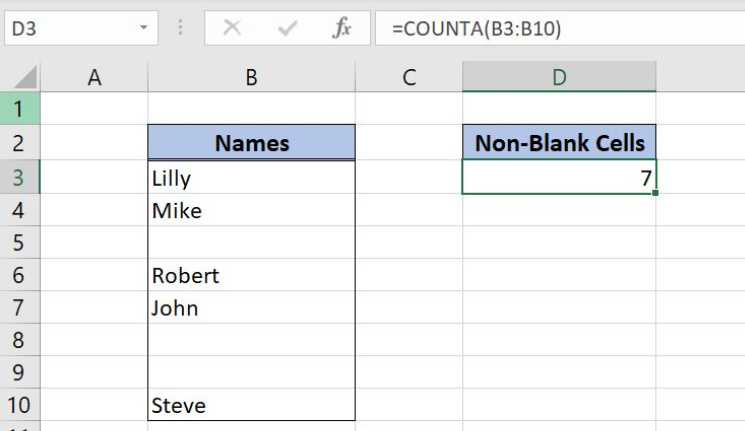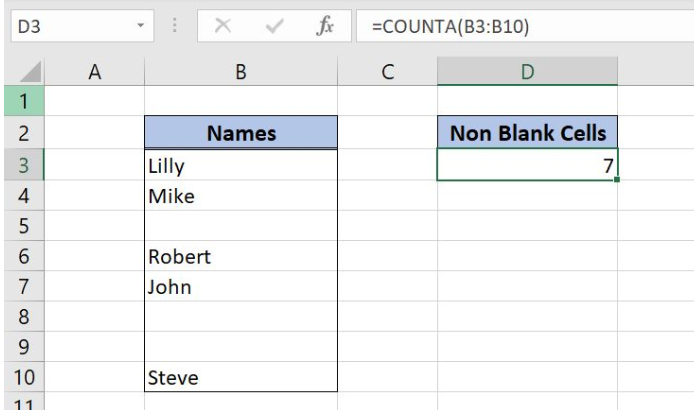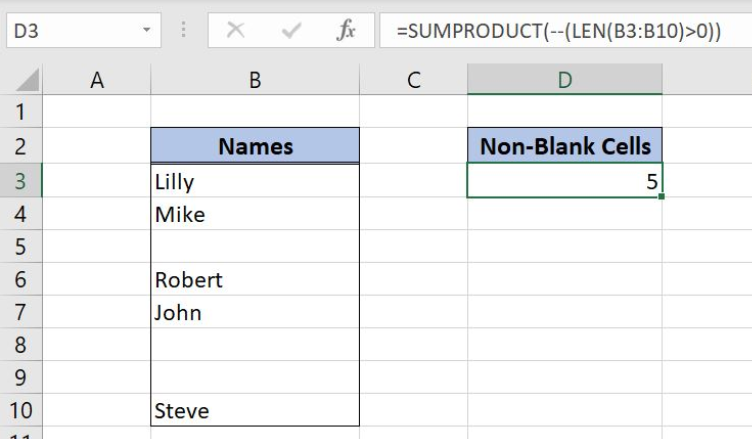Get instant live expert help with Excel or Google Sheets“My Excelchat expert helped me in less than 20 minutes, saving me what would have been 5 hours of work!”

#### Post your problem and you’ll get Expert help in seconds.

Your message must be at least 40 characters
Our professional Expert are available now. Your privacy is guaranteed.

# Count cells that are not blank

Excel allows a user to count all cells that contain any value, by using the COUNTA function.  The function takes in count texts, numbers, spaces, results of other function, etc. This step by step tutorial will assist all levels of Excel users in counting non-blank cells in a range.Figure 1. The result of the COUNTA function

## Syntax of the COUNTA Formula

The generic formula for the COUNTA function is:

`=COUNTA(range)`

The parameter of the COUNTA function is:

• range – a range of cells where we want to count non-blank cells.

## Counting Non-Blank Cells Using the COUNTA Function

In our example, we want to count all non-blank cells in the range B3:B10. In the cell B8, we put a space. In the cell B9, there is the formula which returns a space as a result.

The formula looks like:

`=COUNTA(B3:B10)`

The parameter range is B3:B10, while in the cell D3 we want to get a result of the COUNTA function.

To apply the COUNTA function, we need to follow these steps:

• Select cell D3 and click on it
• Insert the formula: `=COUNTA(B3:B10)`
• Press enterFigure 2. Using the COUNTA function to count non-blank cells in the range

There are 5 names in the range and also two spaces. Because of that, the result of the function in the cell D3 is 7.

## Ignoring Empty Strings When Using the COUNTA Function

The COUNTA function takes in the count all cells that are not blank. This includes spaces and empty strings returned by other function, which can’t be seen in the range. If we want to ignore these empty strings, we can use the SUMPRODUCT function instead. We will show how it works in the same example. The formula looks like:

`=SUMPRODUCT(--(LEN(B3:B10) > 0))`Figure 3. Counting non-blank cells with the SUMPRODUCT function

The function checks all cells in the range if their length is greater than 0. In the end, it counts all cells which are greater than 0. As you can see, the result of the function is 5.

Most of the time, the problem you will need to solve will be more complex than a simple application of a formula or function. If you want to save hours of research and frustration, try our live Excelchat service! Our Excel Experts are available 24/7 to answer any Excel question you may have. We guarantee a connection within 30 seconds and a customized solution within 20 minutes.

### Did this post not answer your question? Get a solution from connecting with the expert.Another blog reader asked this question today on Excelchat:
Solution examplesi have a table with Dates, First names , Last names and i want to count how many times a name occurs, but if a name occurs more than once on a particular date i need to only count it once. this is on excel and not google sheets
Solved by F. H. in 31 minsI need cell B2 Green if cells G2:Q2 are empty. If cells G2:Q2 have any text in any cell then i need B2 to be red.
Solved by C. W. in 20 minsI have a Heading Title at the top of each column and keep my tally (formula) of that column in the second row. However, ever time I sort/filter my worksheet the second row also gets sorted and I lost my tally - which I use for analysis in the second sheet. I have tried freeze and split and neither works. Please help !
Solved by F. Q. in 40 minsHi, Hopefully someone will be able to help me. I'm wanting to calculate the average sum contained within a range of cells discarding blanks. The problem that I'm encountering is that there is an additional feature where I only want to take into consideration columns that contain specific text. =SUMIF(G79:HG79,"Sep-18",(G6:HG6/COUNTA(G6:HG6))) G79:HG79 will contain the specific text with G:HG containing the digits. Cheers all in advance
Solved by M. J. in 20 minsHi - I need a formula that can look at a Member Number (Column A) and report back if location (Column B) matches 1 of 6 locations, then looks to the date of occurance (Column N) but only adds back 1 value of repeating dates. I have this, but it is not coming back with the right answer... =SUMPRODUCT(('2017'!I\$3:I\$10000=\$A223)*ISNUMBER(SEARCH({"2","6","58","69","76","97"},'2017'!N\$3:N\$10000))) Information is corporate private, so I won't be uploading file.
Solved by S. L. in 60 mins## Subscribe to Excelchat.coAnother blog reader asked this question today on Excelchat: